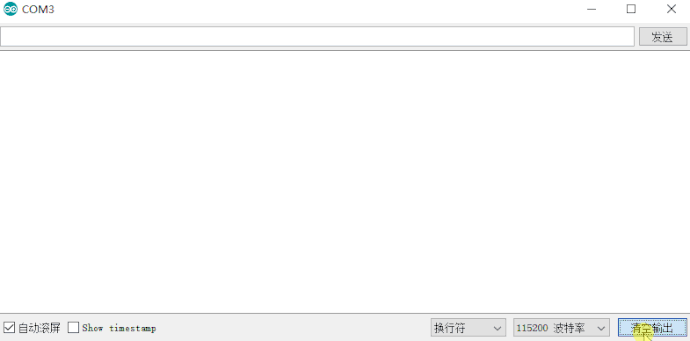# Arduino for BPI:bit 14 : How to use onboard Buzzer

Arduino for BPI:bit 14 : How to use onboard Buzzer,Learn PWM by using the bpi-bit board buzzer.

use BPI:bit + Arduino IDE 1.8.9

mainfunction:

`double ledcSetup(uint8_t channel, double freq, uint8_t resolution_bits)`

Set the frequency and count bits (duty ratio resolution) corresponding to the LEDC channel, and this method returns the final frequency

Channel final frequency = clock/(frequency division coefficient * (1 << count digit)); (maximum frequency division coefficient is 1024)

parameters function
`channel` channel number 0 ~ 15
`freq` Desired setting frequency
`resolution_bits` Number of count, value 0 ~ 20 (this value determines the writable duty ratio in the ledcWrite method later. For example, if the duty ratio is written as 10, the maximum duty ratio can be written as 1023, i.e. (1<< resolution_bit-1).

`void ledcAttachPin(uint8_t pin, uint8_t channel)`Connect the Pin Pin to the channel number

`void ledcWrite(uint8_t channel, uint32_t duty)`Specify the channel output a certain duty ratio waveform

Code instructions

Convert 16-bit PWM to 8-bit precision

``````void ledcAnalogWrite(uint8_t channel, uint32_t value)
{
//value_MAX = 255
uint32_t duty = (65535 / 255) * value;
// write duty to LEDC
ledcWrite(channel, duty);
}

``````

code

To test the code, simply compile it using the Arduino IDE and upload it to the ESP32 development board

``````#include <Arduino.h>

#define LEDC_CHANNEL_0 0
#define LEDC_TIMER_16_BIT 16

#define Buzzer 25

#define brightness 10

int BuzzerTestFreq = 0;
int LEDC_BASE_FREQ = 200;

/*****************************************************************
* Function        :ledcAnalogWrite                              *
* Input Parameter :channel / value                              *
* Output Parameter:None                                         *
* Features        :Match value to channel and convert to duty   *
****************************************************************/
void ledcAnalogWrite(uint8_t channel, uint32_t value)
{
//value_MAX = 255
uint32_t duty = (65535 / 255) * value;
// write duty to LEDC
ledcWrite(channel, duty);
}

void setup()
{
Serial.begin(115200);
}

void loop()
{

ledcSetup(LEDC_CHANNEL_0, LEDC_BASE_FREQ, LEDC_TIMER_16_BIT);
ledcAttachPin(Buzzer, LEDC_CHANNEL_0);
LEDC_BASE_FREQ = LEDC_BASE_FREQ + 10;
// set the brightness on LEDC channel 0
ledcAnalogWrite(LEDC_CHANNEL_0, brightness);
delay(100);
Serial.print("Freq:");
Serial.print(LEDC_BASE_FREQ);
Serial.print("           Duty:");
Serial.print(brightness/2.55);
Serial.println();
ledcAnalogWrite(LEDC_CHANNEL_0, 0);
}
``````

The test results

The buzzer voice will get higher and higher

The serial monitor on the Arduino IDE outputs the following resultsreference docments:

esp32-hal-ledc.h

esp32-hal-ledc.c

ESP32 chip datasheet